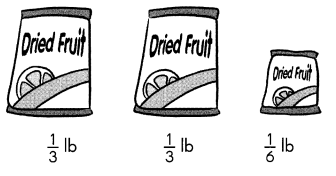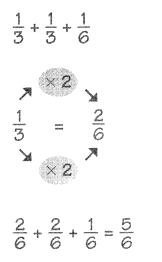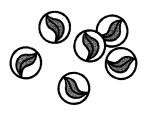# Math in Focus Grade 4 Chapter 6 Practice 8 Answer Key Real-World Problems: Fractions

Practice the problems of Math in Focus Grade 4 Workbook Answer Key Chapter 6 Practice 8 Real-World Problems: Fractions to score better marks in the exam.

## Math in Focus Grade 4 Chapter 6 Practice 8 Answer Key Real-World Problems: Fractions

Example
Ali bought three pockets of dried fruit.What is the total weight of all three packets of dried fruit?The total weight of aB three packets of dried fruit is $$\frac{5}{6}$$ pound.

Question 1.
He ate $$\frac{1}{6}$$ of one waffle, and $$\frac{2}{3}$$ of another waffle.
How many waffles were left?
Amount of waffles were left = 13 ÷ 6 or $$\frac{13}{6}$$

Explanation:
Amount of waffles he ate = $$\frac{1}{6}$$ of one waffle + $$\frac{2}{3}$$ of another waffle.
Amount of waffles were left = (1 – $$\frac{1}{6}$$ ) + (1 – $$\frac{2}{3}$$) + 1
= [(6-1) ÷ 6] + [(3-2) ÷ 3] + 1
= $$\frac{5}{6}$$ + $$\frac{1}{3}$$ + 1
= [(5 + 2) ÷ 6] + 1
= $$\frac{7}{6}$$ + 1
= (7 + 6) ÷ 6
= 13 ÷ 6 or $$\frac{13}{6}$$

Question 2.
A grocery store has 5 pounds of granola. One customer buys $$\frac{2}{3}$$ pound of granola and another buys $$\frac{5}{6}$$ pound. After these purchases, how much granola is left?
Number of pounds left over = 5 ÷ 3 or  $$\frac{5}{3}$$

Explanation:
Number of pounds one customer buys = $$\frac{2}{3}$$  of granola
=> $$\frac{2}{3}$$ × 5
=> $$\frac{10}{3}$$
Number of pounds another customer buys = $$\frac{5}{6}$$
Total number of pounds granola grocery store has = 5.
Total number of pounds granola grocery store has = Number of pounds one customer buys + Number of pounds another customer buys + Number of pounds left over
=> 5 = $$\frac{10}{3}$$ + $$\frac{5}{6}$$ + Number of pounds left over
=> 5 = [(20 + 5) ÷ 6] + Number of pounds left over
=> 5 = $$\frac{10}{3}$$ + Number of pounds left over
=> 5 – $$\frac{10}{3}$$  = Number of pounds left over
=> (15 – 10) ÷ 3 = Number of pounds left over
=> $$\frac{5}{3}$$  or 5 ÷ 3 = Number of pounds left over

Question 3.
Karen jogs $$\frac{1}{2}$$ mile. Selma jogs $$\frac{1}{4}$$ mile more than Karen. Lena jogs $$\frac{3}{4}$$ mile more than Selma. How far does Lena jog?
Number of miles Lena jogs = $$\frac{3}{2}$$

Explanation:
Number of miles Karen jogs = $$\frac{1}{2}$$
Selma jogs $$\frac{1}{4}$$ mile more than Karen.
=> Number of miles Selma jogs = Number of miles Karen jogs + $$\frac{1}{4}$$
= $$\frac{1}{2}$$ + $$\frac{1}{4}$$
= (2 + 1) ÷ 4
= $$\frac{3}{4}$$
Lena jogs $$\frac{3}{4}$$ mile more than Selma.
=> Number of miles Lena jogs = Number of miles Selma jogs + $$\frac{3}{4}$$
= $$\frac{3}{4}$$ + $$\frac{3}{4}$$
= $$\frac{6}{4}$$
= $$\frac{3}{2}$$

Question 4.
Jeremy has 18 marbles. He loses 6 of them.a. What fraction of the marbles does he lose?
Fraction of the marbles he lost = 1 ÷ 3 or $$\frac{1}{3}$$ or 0.33.

Explanation:
Total number of marbles Jeremy has = 18.
Number of marbles he loses = 6.
Fraction of the marbles he lost = Number of marbles he loses ÷ Total number of marbles Jeremy has
= 6 ÷ 18
= 1 ÷ 3 or $$\frac{1}{3}$$ or 0.33.

b. What fraction of the marbles does he have left?
Fraction of the marbles does he have left = 2 ÷ 3 or $$\frac{2}{3}$$ or 0.67.

Explanation:
Total number of marbles Jeremy has = 18.
Number of marbles he loses = 6.
Number of the marbles he have left = Total number of marbles Jeremy has – Number of marbles he loses
= 18 – 6
= 12.
Fraction of the marbles does he have left = Number of the marbles he have left ÷ Total number of marbles Jeremy has
= 12 ÷ 18
= 2 ÷ 3 or $$\frac{2}{3}$$ or 0.67.

Question 5.
Mrs. Yan buys 4 red tulips and 5 yellow tulips.

a. What fraction of the tulips are red?
Fraction of the tulips are red = 4 ÷ 9 or $$\frac{4}{9}$$

Explanation:
Number of red tulips Mrs. Yan buys = 4.
Number of yellow tulips Mrs. Yan buys = 5.
Total number of tulips Mrs. Yan buys = Number of red tulips Mrs. Yan buys + Number of yellow tulips Mrs. Yan buys
= 4 + 5
= 9.
Fraction of the tulips are red = Number of red tulips Mrs. Yan buys ÷ Total number of tulips Mrs. Yan buys
= 4 ÷ 9 or $$\frac{4}{9}$$

b. What fraction of the tulips are yellow?
Fraction of the tulips are yellow = 5 ÷ 9 or $$\frac{5}{9}$$

Explanation:
Number of red tulips Mrs. Yan buys = 4.
Number of yellow tulips Mrs. Yan buys = 5.
Total number of tulips Mrs. Yan buys = Number of red tulips Mrs. Yan buys + Number of yellow tulips Mrs. Yan buys
= 4 + 5
= 9.
Fraction of the tulips are yellow = Number of yellow tulips Mrs. Yan buys ÷ Total number of tulips Mrs. Yan buys
= 5 ÷ 9 or $$\frac{5}{9}$$

Question 6.
Charles owns 3 cats, 4 goldfish, and some parakeets. Altogether, he has 10 pets.

a. What fraction of his pets are goldfish?
Fraction of his pets are goldfish = 2 ÷ 5 or $$\frac{2}{5}$$

Explanation:
Number of cats Charles owns = 3.
Number of goldfish Charles owns = 4.
Total number of pets Charles owns = 10.
Fraction of his pets are goldfish = Number of goldfish Charles owns ÷ Total number of pets Charles owns
= 4 ÷ 10
= 2 ÷ 5 or $$\frac{2}{5}$$

b. What fraction of his pets are parakeets?
Fraction of his pets are parakeets = 3 ÷ 10 or $$\frac{3}{10}$$

Explanation:
Number of cats Charles owns = 3.
Number of goldfish Charles owns = 4.
Total number of pets Charles owns = 10.
=> Total number of pets Charles owns  = Number of parakeets Charles owns + Number of cats Charles owns + Number of goldfish Charles owns
=> 10 = ?? + 3 + 4
=> 10 = ?? + 7
=> 10 – 7 = ??
=> 3 = ??.
Fraction of his pets are parakeets = Number of parakeets Charles owns ÷ Total number of pets Charles owns
= 3 ÷ 10 or $$\frac{3}{10}$$

Question 7.
Rick had $20. He spent$10 on food, $6 on a movie ticket and saved the rest. a. How much money did he save? Answer: Amount of money he saved =$4.

Explanation:
Total amount of money Rick had = $20. Amount of money Rick had spent on food =$10.
Amount of money Rick had spent on a movie ticket = $6. Amount of money he saved = Total amount of money Rick had – (Amount of money Rick had spent on food + Amount of money Rick had spent on a movie ticket) =$20 – ($10 +$6)
= $20 –$16
= $4. b. What fraction of the total amount did he save? Answer: Fraction of the total amount did he save = 1 ÷ 5 or $$\frac{1}{5}$$ Explanation: Total amount of money Rick had =$20.
Amount of money he saved = \$4.
Fraction of the total amount did he save = Amount of money he saved ÷ Total amount of money Rick had
= 4 ÷ 20
= 1 ÷ 5 or $$\frac{1}{5}$$

Question 8.
There are 24 students in a class, and $$\frac{2}{3}$$ of the students in the class are boys. How many students are girls?
Number of girls in the class = 8.

Explanation:
Total number of students in the class = 24.
$$\frac{2}{3}$$ of the students in the class are boys.
=> Number of boys in the class = Number of students in the class × $$\frac{2}{3}$$
= 24 × $$\frac{2}{3}$$
= 8 × 2
= 16.
Number of girls in the class = Total number of students in the class – Number of boys in the class
= 24 – 16
= 8.

Question 9.
One morning, The Shirt Shop sold 15 T-shirts.
Of the T-shirts sold, $$\frac{1}{5}$$ were gray. The rest were white. How many white T-shirts were sold?
Number of white T-shirts were sold = 12.

Explanation:
Number of T-shirts The Shirt Shop sold One morning = 15.
Of the T-shirts sold, $$\frac{1}{5}$$ were gray.
=> Number of gray T-shirts sold = Number of T-shirts The Shirt Shop sold One morning × $$\frac{1}{5}$$
= 15 × $$\frac{1}{5}$$
= 3 × 1
= 3.
Number of white T-shirts were sold = Number of T-shirts The Shirt Shop sold One morning – Number of gray T-shirts sold
= 15 – 3
= 12.

Question 10.
A chef bought some green and red peppers. She bought 18 green peppers, which was $$\frac{3}{4}$$ of the total number.

a. How many red peppers did she buy?
Number of red peppers she buys = 6.

Explanation:
Number of green peppers She bought = 18.
She bought 18 green peppers, which was $$\frac{3}{4}$$ of the total number.
Let the total number of peppers she buys be X.
Number of green peppers She bought = Total number of peppers she buys × $$\frac{3}{4}$$
= 18 = X × $$\frac{3}{4}$$
=> 18 × $$\frac{4}{3}$$ = X
=> 6 × 4 = X
=> 24 = X.
Number of red peppers she buys = Total number of peppers she buys – Number of green peppers She bought
= 24 – 18
= 6.

b. How many peppers did she buy altogether?
Total number of peppers she buys = 24.

Explanation:
Number of green peppers She bought = 18.
She bought 18 green peppers, which was $$\frac{3}{4}$$ of the total number.
Let the total number of peppers she buys be X.
Number of green peppers She bought = Total number of peppers she buys × $$\frac{3}{4}$$
= 18 = X × $$\frac{3}{4}$$
=> 18 × $$\frac{4}{3}$$ = X
=> 6 × 4 = X
=> 24 = X.

Question 11.
There were 25 melons in a box at the grocery store.
The store sold $$\frac{3}{5}$$ of the melons.
How many melons were sold?
Number of melons sold = 15.

Explanation:
Number of melons in a box at the grocery store = 25.
The store sold $$\frac{3}{5}$$ of the melons.
=> Number of melons sold = Number of melons in a box at the grocery store × $$\frac{3}{5}$$
= 25 × $$\frac{3}{5}$$
= 5 × 3
= 15.

Question 12.
Ava read $$\frac{1}{4}$$ of a book on Monday, and $$\frac{1}{5}$$ of it on Tuesday. There are 80 pages in the book. How many pages did she read altogether on both days?
Number of pages she read altogether on both days = 36.

Explanation:
Number of pages in the book = 80.
Ava read $$\frac{1}{4}$$ of a book on Monday.
=> Number of pages Ava read on Monday = Number of pages in the book × $$\frac{1}{4}$$
= 80 × $$\frac{1}{4}$$
= 20 × 1
= 20.
Ava read $$\frac{1}{5}$$ of it on Tuesday.
> Number of pages Ava read on Tuesday = Number of pages in the book × $$\frac{1}{5}$$
= 80 × $$\frac{1}{5}$$
= 16 × 1
= 16.
Number of pages she read altogether on both days = Number of pages Ava read on Monday + Number of pages Ava read on Tuesday
= 20 + 16
= 36.